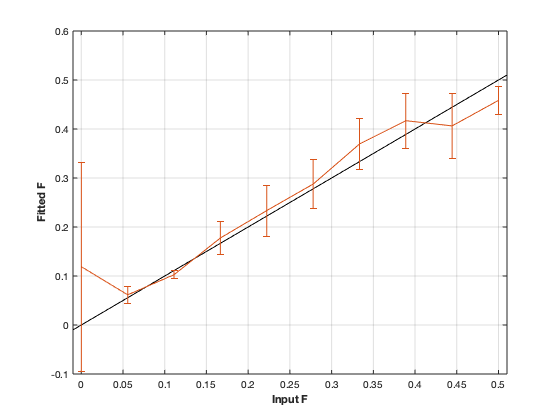# qmt_spgr: quantitative Magnetizatoion Transfer (qMT) using Spoiled Gradient Echo (or FLASH)¶## Contents

```% This m-file has been automatically generated using qMRgenBatch(qmt_spgr)
% Command Line Interface (CLI) is well-suited for automatization
% purposes and Octave.
%
% Please execute this m-file section by section to get familiar with batch
% processing for qmt_spgr on CLI.
%
%
% Written by: Agah Karakuzu, 2017
% =========================================================================
```

## I- DESCRIPTION

```qMRinfo('qmt_spgr'); % Describe the model
```
``` qmt_spgr:  quantitative Magnetizatoion Transfer (qMT) using Spoiled Gradient Echo (or FLASH)
a href="matlab: figure, imshow qmt_spgr.png ;"Pulse Sequence Diagram/a

Assumptions:
FILL

Inputs:
MTdata              Magnetization Transfert data
(R1map)             1/T1map (VFA RECOMMENDED Boudreau 2017 MRM)
(B1map)             B1 field map, used for flip angle correction (=1 if not provided)
(B0map)             B0 field map, used for offset correction (=0Hz if not provided)

Outputs:
F                   Ratio of number of restricted pool to free pool, defined
as F = M0r/M0f = kf/kr.
kr                  Exchange rate from the free to the restricted pool
(note that kf and kr are related to one another via the
definition of F. Changing the value of kf will change kr
accordingly, and vice versa).
R1f                 Longitudinal relaxation rate of the free pool
(R1f = 1/T1f).
R1r                 Longitudinal relaxation rate of the restricted pool
(R1r = 1/T1r).
T2f                 Tranverse relaxation time of the free pool (T2f = 1/R2f).
T2r                 Tranverse relaxation time of the restricted pool (T2r = 1/R2r).
(kf)                Exchange rate from the restricted to the free pool.
(resnorm)           Fitting residual.

Protocol:
MTdata              Array [Nb of volumes x 2]
Angle             MT pulses angles (degree)
Offset            Offset frequencies (Hz)

TimingTable         Vector [5x1]
Tmt               Duration of the MT pulses (s)
Ts                Free precession delay between the MT and excitation pulses (s)
Tp                Duration of the excitation pulse (s)
Tr                Free precession delay after the excitation pulse, before
the next MT pulse (s)
TR                Repetition time of the whole sequence (TR = Tmt + Ts + Tp + Tr)

Options:
MT Pulse
Shape                 Shape of the MT pulse.
Available shapes are:
- hard
- gaussian
- gausshann (gaussian pulse with Hanning window)
- sinc
- sinchann (sinc pulse with Hanning window)
- singauss (sinc pulse with gaussian window)
- fermi
Sinc TBW              Time-bandwidth product for the sinc MT pulses
(applicable to sinc, sincgauss, sinchann MT
pulses).
Bandwidth             Bandwidth of the gaussian MT pulse (applicable
to gaussian, gausshann and sincgauss MT pulses).
Fermi transition (a)  slope 'a' (related to the transition width)
of the Fermi pulse (applicable to fermi MT
pulse).
Assuming pulse duration at 60 dB (from the Bernstein handbook)
and t0 = 10a,
slope = Tmt/33.81;
# of MT pulses        Number of pulses used to achieve steady-state
Fitting constraints
Use R1map to         By checking this box, you tell the fitting
constrain R1f          algorithm to check for an observed R1map and use
its value to constrain R1f. Checking this box
will automatically set the R1f fix box to true
in the Fit parameters table.
Fix R1r = R1f        By checking this box, you tell the fitting
algorithm to fix R1r equal to R1f. Checking this
box will automatically set the R1r fix box to
true in the Fit parameters table.
Fix R1f*T2f          By checking this box, you tell the fitting
algorithm to compute T2f from R1f value. R1f*T2f
value is set in the next box.
R1f*T2f =            Value of R1f*T2f (no units)

Model                  Model you want to use for fitting.
Available models are:
- SledPikeRP (Sled  Pike rectangular pulse),
- SledPikeCW (Sled  Pike continuous wave),
- Yarkykh (Yarnykh  Yuan)
- Ramani
Note: Sled  Pike models will show different
options than Yarnykh or Ramani.
Lineshape              The absorption lineshape of the restricted pool.
Available lineshapes are:
- Gaussian
- Lorentzian
- SuperLorentzian
Read pulse alpha       Flip angle of the excitation pulse.
Compute SfTable        By checking this box, you compute a new SfTable

Command line usage:
a href="matlab: qMRusage(qmt_spgr);"qMRusage(qmt_spgr/a

Author: Ian Gagnon, 2017

References:
Please cite the following if you use this module:
Sled, J.G., Pike, G.B., 2000. Quantitative interpretation of magnetization transfer in spoiled gradient echo MRI sequences. J. Magn. Reson. 145, 24?36.
In addition to citing the package:
Karakuzu A., Boudreau M., Duval T.,Boshkovski T., Leppert I.R., Cabana J.F.,
Gagnon I., Beliveau P., Pike G.B., Cohen-Adad J., Stikov N. (2020), qMRLab:
Quantitative MRI analysis, under one umbrella doi: 10.21105/joss.02343

Reference page in Doc Center
doc qmt_spgr

```

## a- create object

```Model = qmt_spgr;
```

## b- modify options

```         |- This section will pop-up the options GUI. Close window to continue.
|- Octave is not GUI compatible. Modify Model.options directly.```
```Model = Custom_OptionsGUI(Model); % You need to close GUI to move on.
```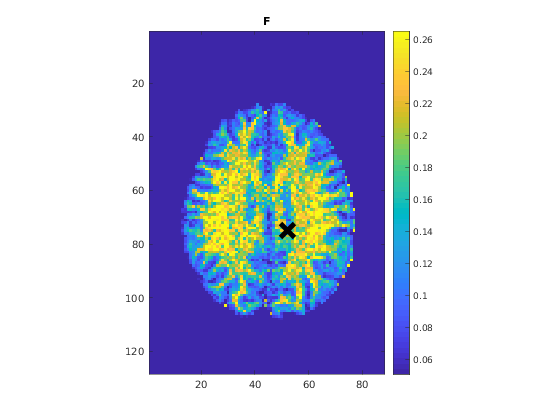## III- FIT EXPERIMENTAL DATASET

```         |- qmt_spgr object needs 5 data input(s) to be assigned:
|-   MTdata
|-   R1map
|-   B1map
|-   B0map
```data = struct();

% MTdata.mat contains [88  128    1   10] data.
% R1map.mat contains [88  128] data.
% B1map.mat contains [88  128] data.
% B0map.mat contains [88  128] data.
% Mask.mat contains [88  128] data.
data.MTdata= double(MTdata);
data.R1map= double(R1map);
data.B1map= double(B1map);
data.B0map= double(B0map);
```

## b- fit dataset

`           |- This section will fit data.`
```FitResults = FitData(data,Model,0);
```
```=============== qMRLab::Fit ======================
Operation has been started: qmt_spgr
Warning: No MToff (i.e. no volumes acquired with Angles=0) -- Fitting assumes
Warning: No MToff (i.e. no volumes acquired with Angles=0) -- Fitting assumes
Warning: No MToff (i.e. no volumes acquired with Angles=0) -- Fitting assumes
Elapsed time is 2.358713 seconds.
Operation has been completed: qmt_spgr
==================================================
```

## c- show fitting results

```         |- Output map will be displayed.
|- If available, a graph will be displayed to show fitting in a voxel.
|- To make documentation generation and our CI tests faster for this model,
we used a subportion of the data (40X40X40) in our testing environment.
|- Therefore, this example will use FitResults that comes with OSF data for display purposes.
|- Users will get the whole dataset (384X336X224) and the script that uses it for demo
via qMRgenBatch(qsm_sb) command.```
```FitResults_old = load('FitResults/FitResults.mat');
qMRshowOutput(FitResults_old,data,Model);
```
```Warning: No MToff (i.e. no volumes acquired with Angles=0) -- Fitting assumes
```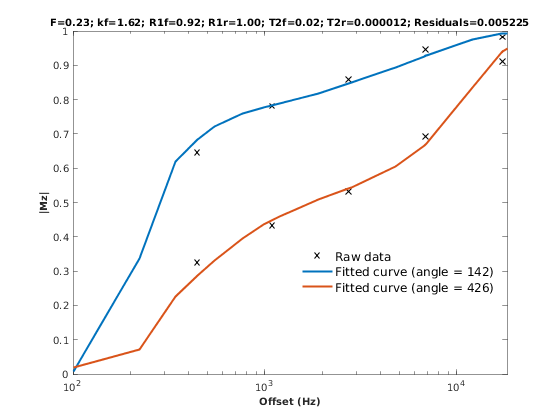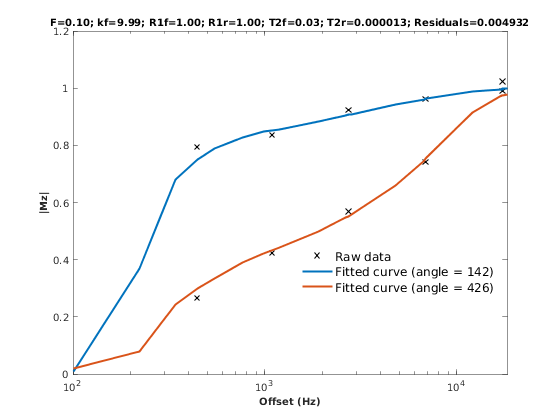## d- Save results

```         |-  qMR maps are saved in NIFTI and in a structure FitResults.mat
that can be loaded in qMRLab graphical user interface
|-  Model object stores all the options and protocol.
It can be easily shared with collaborators to fit their
own data or can be used for simulation.```
```FitResultsSave_nii(FitResults);
Model.saveObj('qmt_spgr_Demo.qmrlab.mat');
```
```Warning: Directory already exists.
```

## V- SIMULATIONS

`   |- This section can be executed to run simulations for qmt_spgr.`

## a- Single Voxel Curve

```         |- Simulates Single Voxel curves:
(1) use equation to generate synthetic MRI data
(3) fit and plot curve```
```      x = struct;
x.F = 0.16;
x.kr = 30;
x.R1f = 1;
x.R1r = 1;
x.T2f = 0.03;
x.T2r = 1.3e-05;
% Set simulation options
Opt.SNR = 50;
Opt.Method = 'Analytical equation';
Opt.ResetMz = false;
% run simulation
figure('Name','Single Voxel Curve Simulation');
FitResult = Model.Sim_Single_Voxel_Curve(x,Opt);
```
```Warning: No MToff (i.e. no volumes acquired with Angles=0) -- Fitting assumes
Warning: No MToff (i.e. no volumes acquired with Angles=0) -- Fitting assumes
```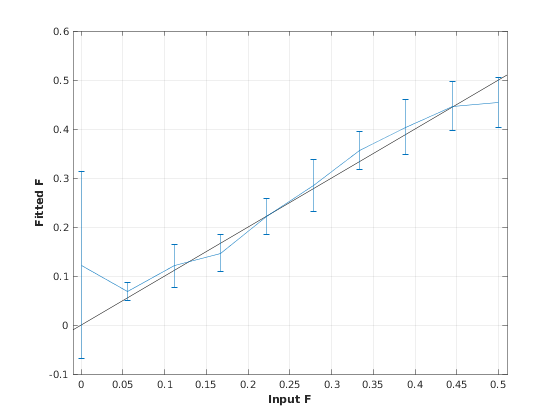## b- Sensitivity Analysis

```         |-    Simulates sensitivity to fitted parameters:
(1) vary fitting parameters from lower (lb) to upper (ub) bound.
(2) run Sim_Single_Voxel_Curve Nofruns times
(3) Compute mean and std across runs```
```      %              F             kr            R1f           R1r           T2f           T2r
OptTable.st = [0.16          30            1             1             0.03          1.3e-05]; % nominal values
OptTable.fx = [0             1             1             1             1             1]; %vary F...
OptTable.lb = [0.0001        0.0001        0.05          0.05          0.003         3e-06]; %...from 0.0001
OptTable.ub = [0.5           1e+02         5             5             0.5           5e-05]; %...to 0.5
% Set simulation options
Opt.SNR = 50;
Opt.Method = 'Analytical equation';
Opt.ResetMz = false;
Opt.Nofrun = 5;
% run simulation
SimResults = Model.Sim_Sensitivity_Analysis(OptTable,Opt);
figure('Name','Sensitivity Analysis');
SimVaryPlot(SimResults, 'F' ,'F' );
```
```Warning: No MToff (i.e. no volumes acquired with Angles=0) -- Fitting assumes
Warning: No MToff (i.e. no volumes acquired with Angles=0) -- Fitting assumes
Warning: No MToff (i.e. no volumes acquired with Angles=0) -- Fitting assumes
Warning: No MToff (i.e. no volumes acquired with Angles=0) -- Fitting assumes
Warning: No MToff (i.e. no volumes acquired with Angles=0) -- Fitting assumes
Warning: No MToff (i.e. no volumes acquired with Angles=0) -- Fitting assumes
Warning: No MToff (i.e. no volumes acquired with Angles=0) -- Fitting assumes
Warning: No MToff (i.e. no volumes acquired with Angles=0) -- Fitting assumes
Warning: No MToff (i.e. no volumes acquired with Angles=0) -- Fitting assumes
Warning: No MToff (i.e. no volumes acquired with Angles=0) -- Fitting assumes
Warning: No MToff (i.e. no volumes acquired with Angles=0) -- Fitting assumes
Warning: No MToff (i.e. no volumes acquired with Angles=0) -- Fitting assumes
Warning: No MToff (i.e. no volumes acquired with Angles=0) -- Fitting assumes
Warning: No MToff (i.e. no volumes acquired with Angles=0) -- Fitting assumes
Warning: No MToff (i.e. no volumes acquired with Angles=0) -- Fitting assumes
Warning: No MToff (i.e. no volumes acquired with Angles=0) -- Fitting assumes
Warning: No MToff (i.e. no volumes acquired with Angles=0) -- Fitting assumes
Warning: No MToff (i.e. no volumes acquired with Angles=0) -- Fitting assumes
Warning: No MToff (i.e. no volumes acquired with Angles=0) -- Fitting assumes
Warning: No MToff (i.e. no volumes acquired with Angles=0) -- Fitting assumes
Warning: No MToff (i.e. no volumes acquired with Angles=0) -- Fitting assumes
Warning: No MToff (i.e. no volumes acquired with Angles=0) -- Fitting assumes
Warning: No MToff (i.e. no volumes acquired with Angles=0) -- Fitting assumes
Warning: No MToff (i.e. no volumes acquired with Angles=0) -- Fitting assumes
Warning: No MToff (i.e. no volumes acquired with Angles=0) -- Fitting assumes
Warning: No MToff (i.e. no volumes acquired with Angles=0) -- Fitting assumes
Warning: No MToff (i.e. no volumes acquired with Angles=0) -- Fitting assumes
Warning: No MToff (i.e. no volumes acquired with Angles=0) -- Fitting assumes
Warning: No MToff (i.e. no volumes acquired with Angles=0) -- Fitting assumes
Warning: No MToff (i.e. no volumes acquired with Angles=0) -- Fitting assumes
Warning: No MToff (i.e. no volumes acquired with Angles=0) -- Fitting assumes
Warning: No MToff (i.e. no volumes acquired with Angles=0) -- Fitting assumes
Warning: No MToff (i.e. no volumes acquired with Angles=0) -- Fitting assumes
Warning: No MToff (i.e. no volumes acquired with Angles=0) -- Fitting assumes
Warning: No MToff (i.e. no volumes acquired with Angles=0) -- Fitting assumes
Warning: No MToff (i.e. no volumes acquired with Angles=0) -- Fitting assumes
Warning: No MToff (i.e. no volumes acquired with Angles=0) -- Fitting assumes
Warning: No MToff (i.e. no volumes acquired with Angles=0) -- Fitting assumes
Warning: No MToff (i.e. no volumes acquired with Angles=0) -- Fitting assumes
Warning: No MToff (i.e. no volumes acquired with Angles=0) -- Fitting assumes
Warning: No MToff (i.e. no volumes acquired with Angles=0) -- Fitting assumes
Warning: No MToff (i.e. no volumes acquired with Angles=0) -- Fitting assumes
Warning: No MToff (i.e. no volumes acquired with Angles=0) -- Fitting assumes
Warning: No MToff (i.e. no volumes acquired with Angles=0) -- Fitting assumes
Warning: No MToff (i.e. no volumes acquired with Angles=0) -- Fitting assumes